# Texas Go Math Grade 8 Lesson 1.1 Answer Key Rational and Irrational Numbers

Refer to our Texas Go Math Grade 8 Answer Key Pdf to score good marks in the exams. Test yourself by practicing the problems from Texas Go Math Grade 8 Lesson 1.1 Answer Key Rational and Irrational Numbers.

## Texas Go Math Grade 8 Lesson 1.1 Answer Key Rational and Irrational Numbers

Write each fraction as a decimal.

Question 1.
$$\frac{5}{11}$$__________
To write $$\frac{5}{11}$$ as a decimal, we divide the numerator by the denominator until the remainder is zero or until the digits in the quotient begin to repeat.
We add as many zeros after the decimal point in the dividend as needed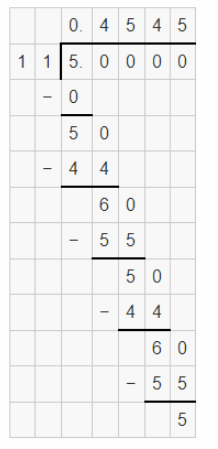When a decimal has one or more digits that repeat indefinitely, we write the decimal with a bar over the repeating digit(s). In our case, 45 repeats indefinitely.
$$\frac{5}{11}$$ = $$0 . \overline{45}$$

Rational and Irrational Numbers Answer Key Question 2.
$$\frac{1}{8}$$ __________
To write $$\frac{1}{8}$$ as a decimal, we divide the numerator by the denominator until the remainder is zero or until the digits in the quotient begin to repeat.
We add as many zeros after the decimal point in the dividend as needed$$\frac{1}{8}$$ = 0.125

Question 3.
2$$\frac{1}{3}$$ _______
First, we convert the mixed number to an improper fraction:
2$$\frac{1}{3}$$ = 2 + $$\frac{1}{3}$$
= $$\frac{6}{3}$$ + $$\frac{1}{3}$$
= $$\frac{7}{3}$$
To write $$\frac{7}{3}$$ as a decimal, we divide the numerator by the denominator until the remainder is zero or until the digits in the quotient begin to repeat.
We add as many zeros after the decimal point in the dividend as needed.When a decimal has one or more digits that repeat indefinitely, we write the decimal with a bar over the repeating digit(s). In our case, 3 represents indefinitely.
2$$\frac{1}{3}$$ = $$2 . \overline{3}$$

Reflect

Question 4.
Analyze Relationships How are the two square roots of a positive number related? Which is the principal square root?
A square root of a number b is the solution of the equation x²=b. It is a number that when multiplied by itself gives you b. Every positive number b has two square roots, denoted √b and √b. The principal square root of b is the positive square root, denoted √bQuestion 5.
Is the principal square root of 2 a whole number? What types of numbers have whole number square roots?
Because √2 is not an integer (2 is not a perfect square), √2 must therefore be irrational. Numbers whose square roots are whole numbers, (or more accurately positive integers) are called perfect square numbers. Numbers with decimals aren’t perfect square roots.

Find the two square roots of each number.

Question 6.
64 ____
The given number is: 64
Now,
We know that,
A square root of a number b is the solution of the equation x²=b. It is a number that when multiplied by itself gives you b. Every positive number b has two square roots, denoted √b and √b
So,
√x = ±b
So,
$$\sqrt{64}$$ = ±8
Hence, from the above,
We can conclude that
$$\sqrt{64}$$ = 8
$$\sqrt{64}$$ = -8

Question 7.
100 _________
The given number is: 100
Now,
We know that,
A square root of a number b is the solution of the equation x²=b. It is a number that when multiplied by itself gives you b. Every positive number b has two square roots, denoted √b and √b
So,
√x = ±b
So,
$$\sqrt{100}$$ = ±10
Hence, from the above,
We can conclude that
$$\sqrt{100}$$ = 10
$$\sqrt{100}$$ = -10

Lesson 1.1 Define Rational Numbers Answer Key Question 8.
$$\frac{1}{9}$$ _________
The given number is: $$\frac{1}{9}$$
Now,
We know that,
A square root of a number b is the solution of the equation x²=b. It is a number that when multiplied by itself gives you b. Every positive number b has two square roots, denoted √b and √b
So,
√x = ±b
Now,
We know that,
$$\sqrt{\frac{a}{b}}$$ = $$\frac{\sqrt{a}}{\sqrt{b}}$$
So,
$$\sqrt{\frac{1}{9}}$$ = $$\frac{\sqrt{1}}{\sqrt{9}}$$
= ±$$\frac{1}{3}$$
Hence, from the above,
We can conclude that
$$\sqrt{\frac{1}{9}}$$ = $$\frac{1}{3}$$
$$\sqrt{\frac{1}{9}}$$ = –$$\frac{1}{3}$$

Question 9.
A square garden has an area of 144 square feet. How long is each side?
It is given that
A square garden has an area of 144 square feet
Now,
We know that,
A square root of a number b is the solution of the equation x²=b. It is a number that when multiplied by itself gives you b. Every positive number b has two square roots, denoted √b and √b
So,
√x = ±b
Now,
We know that,
The area of a square = Side²
So,
Side² = 144
$$\sqrt{Side²}$$ = $$\sqrt{144}$$
Side = ± 12
But,
We know that,
The side of a square will not be negative
So,
The side of a square field is: 12 feet
Hence, from the above,
We can conclude that
The side of a square field is: 12 feet

Explore Activity 1
Estimating Irrational Numbers
Irrational numbers are numbers that are not rational. in other words, they cannot be written in the form $$\frac{a}{b}$$ where a and b are integers and b is not 0.

Estimate the value of $$\sqrt{2}$$.

A. Since 2 is not a perfect square, $$\sqrt{2}$$ is irrational.
B. To estimate $$\sqrt{2}$$, first find two consecutive perfect squares that 2 is between. Complete the inequality by writing these perfect squares in the boxes.
1 < 2 < 9
C. Now take the square root of each number.
$$\sqrt{1}$$ < $$\sqrt{2}$$ < $$\sqrt{9}$$
D. Simplify the square roots of perfect squares.
$$\sqrt{2}$$ is between _____ and ___.
1 < $$\sqrt{2}$$ < 3
E. Estimate that $$\sqrt{2}$$ ≈ 1.5.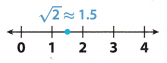F. To find a better estimate, first, choose some numbers between 1 and 2 and square them. For example, choose 1.3, 1.4, and 1.5.
1.32 = 1.69
1.42 = 1.96
1.52 = 2.25
Is $$\sqrt{2}$$ between 1.3 and 1.4? How do you know?
From the above,
We can observe that
The estimation of $$\sqrt{2}$$ is: 1.5
Now,
From part (f),
We can observe that
The squares of the numbers 1.3, 1.4, and 1.5
Hence, from the above,
We can observe that
$$\sqrt{2}$$ is not between 1.3 and 1.4

Is $$\sqrt{2}$$ between 1.4 and 1.5? How do you know?
From the above,
We can observe that
The estimation of $$\sqrt{2}$$ is: 1.5
Now,
From part (f),
We can observe that
The squares of the numbers 1.3, 1.4, and 1.5
Hence, from the above,
We can observe that
$$\sqrt{2}$$ is not between 1.4 and 1.5
So,
$$\sqrt{2}$$ is between 1.4 and 1.5.
So,
$$\sqrt{2}$$ ≈ 1.5
G. Locate and label this value on the number line.Reflect

Question 10.
How could you find an even better estimate of $$\sqrt{2}$$?
The given number is: $$\sqrt{2}$$
Now,
Find the perfect squares that is between $$\sqrt{2}$$
So,
1 < $$\sqrt{2}$$ < 3
We know that,
$$\sqrt{2}$$ ≈ 1.414
So,
The value of $$\sqrt{2}$$ lies closer to: 1.4
Hence, from the above,
We can conclude that
The better estimate of $$\sqrt{2}$$ is: 1.4Question 11.
Find a better estimate of $$\sqrt{2}$$. Draw a number line and locate and label your estimate.$$\sqrt{2}$$ is between _____ and _____, so $$\sqrt{2}$$ ≈ ___
The given number is: $$\sqrt{2}$$
Now,
Find the perfect squares that is between $$\sqrt{2}$$
So,
1 < $$\sqrt{2}$$ < 3
So,
The value of $$\sqrt{2}$$ lies between 1.4 and 1.5
Now,
We know that,
$$\sqrt{2}$$ ≈ 1.414
So,
The value of $$\sqrt{2}$$ lies closer to: 1.4
Now,
The representation of the value of $$\sqrt{2}$$ on a number line is:Hence, from the above,
We can conclude that
$$\sqrt{2}$$ is between 1.4 and 1.5,
So,
$$\sqrt{2}$$ ≈ 1.5

Question 12.
Estimate the value of $$\sqrt{7}$$ to the nearest 0.05. Draw a number line and locate and label your estimate.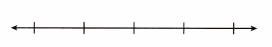$$\sqrt{7}$$ is between and _____, so $$\sqrt{7}$$ ≈ ___.
The given number is: $$\sqrt{7}$$
Now,
Find the perfect squares that lies between 7
So,
4 < 7 < 9
$$\sqrt{4}$$ < $$\sqrt{7}$$ < $$\sqrt{9}$$
2 < $$\sqrt{7}$$ < 3
Now,
2.1² = 4.41
2.5²= 6.25
2.6² = 6.76
Now,
From the above,
We can observe that
The Estimate of $$\sqrt{7}$$ is approximately equal to: 6.76
Now,
The representation of $$\sqrt{7}$$ on a number line is: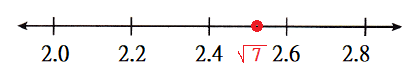Hence, from the above,
We can conclude that
$$\sqrt{7}$$ is between 2.5 and 2.6
So,
$$\sqrt{7}$$ ≈ 2.6

Explore Activity 2
Approximating π
The number π, the ratio of the circumference of a circle to its diameter, is an irrational number. It cannot be written as the ratio of two integers.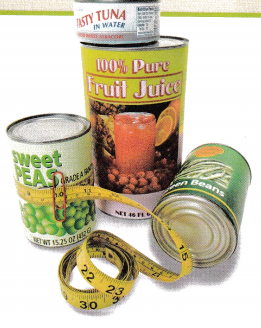In this activity, you will explore the relationship between the diameter and circumference of a circle.
A. Use a tape measure to measure the circumference and the diameter of four circular objects using metric measurements. To measure the circumference, wrap the tape measure tightly around the object and determine the mark where the tape starts to overlap the beginning of the tape. When measuring the diameter, be sure to measure the distance across the object at its widest point.
The examples of the four circular objects are:
a. CD
b. Bottle lid
c. Frisbee
d. Bowl

B. Record the circumference and diameter of each object in the table.C. Divide the circumference by the diameter for each object. Round each answer to the nearest hundredth and record it in the table.D. Describe what you notice about the ratio of circumference to diameter.
From part (b),
The complete table is:Now,
From the above table,
We can observe that
The ratio of the circumference to diameter is approximately equal to 3
Hence, from the above,
We can conclude that
The point we noticed is that
The ratio of the circumference to diameter is approximately equal to 3

Reflect

Lesson 1.1 Rational Numbers as Decimals Answer Key Question 13.
What does the fact that π is irrational indicate about its decimal equivalent?
π is an irrational number because its decimal expansion is a “Non-Terminating Non-Recurring decimal”

Question 14.
Plot π on the number line.We know that,
The value of π is: 3.14
Hence, from the above,
We can conclude that
The representation of π on the number line is:Question 15.
Explain Why A CD and a DVD have the same diameter. Explain why they have the same circumference.
It is given that
A CD and a DVD have the same diameter
Now,
We know that,
The shapes of CD and DVDs are in circular shape
Now,
We know that,
The circumference of a circle (C) = πd
Where,
d is the diameter
It is given that
The diameter is the same for both CD and DVD
So,
The circumference will also be the same since the value of π is constant
Hence, from the above,
We can conclude that
The CD and DVD have the same circumference since the values of diameter and π are constant

Question 1.
Vocabulary Square roots of numbers that are not perfect squares are ____________
We know that,
The square roots of numbers that are not perfect squares are called “Irrational Numbers”
Hence, from the above,
We can conclude that
The term that best suits the given statement is: irrational Numbers

Write each fraction as a decimal. (Example 1)

$$\frac{7}{8}$$ ____
The given fraction is: $$\frac{7}{8}$$
Now,
By using the Long Division,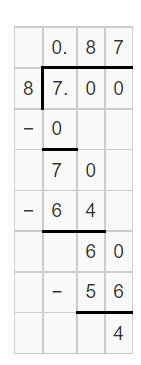Hence, from the above,
We can conclude that
The representation of $$\frac{7}{8}$$ as a decimal is: 0.87

Question 3.
$$\frac{17}{20}$$ ________
The given fraction is: $$\frac{17}{20}$$
Now,
By using the Long Division,Hence, from the above,
We can conclude that
The representation of $$\frac{17}{20}$$ as a decimal is: 0.85

Question 4.
$$\frac{18}{25}$$ ________
The given fraction is: $$\frac{18}{25}$$
Now,
By using the Long Division,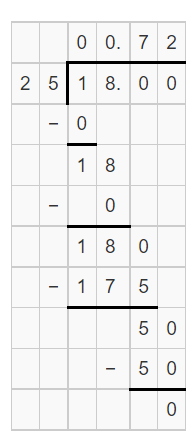Hence, from the above,
We can conclude that
The representation of $$\frac{18}{25}$$ as a decimal is: 0.72

Go Math Grade 8 Lesson 1.1 Question 5.
2$$\frac{3}{8}$$ ________
The given mixed number is: 2$$\frac{3}{8}$$
Now,
The representation of the given mixed number in the form of a fraction is: $$\frac{19}{8}$$
Now,
By using the Long Division,Hence, from the above,
We can conclude that
The representation of $$\frac{19}{8}$$ as a decimal is: 0.42

Question 6.
5$$\frac{2}{3}$$ ________
The given mixed number is: 5$$\frac{2}{3}$$
Now,
The representation of the given mixed number in the form of a fraction is: $$\frac{17}{3}$$
Now,
By using the Long Division,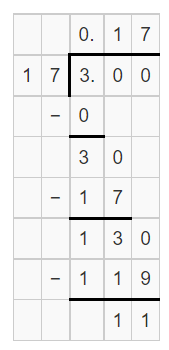Hence, from the above,
We can conclude that
The representation of $$\frac{17}{3}$$ as a decimal is: 0.17

Question 7.
2$$\frac{4}{5}$$ ________
The given mixed number is: 2$$\frac{4}{5}$$
Now,
The representation of the given mixed number in the form of a fraction is: $$\frac{14}{5}$$
Now,
By using the Long Division,Hence, from the above,
We can conclude that
The representation of $$\frac{14}{5}$$ as a decimal is: 0.35

Find the two square roots of each number. (Example 2)

Question 8.
49 ______________
The given number is: 49
Now,
We know that,
A square root of a number b is the solution of the equation x²=b. It is a number that when multiplied by itself gives you b. Every positive number b has two square roots, denoted √b and √b
So,
√x = ±b
So,
$$\sqrt{49}$$ = ±7
Hence, from the above,
We can conclude that
$$\sqrt{49}$$ = 7
$$\sqrt{49}$$ = -7

Go Math Grade 8 Chapter 1 Lesson 1.1 Question 9.
144 _____________
The given number is: 144
Now,
We know that,
A square root of a number b is the solution of the equation x²=b.
It is a number that when multiplied by itself gives you b.
Every positive number b has two square roots, denoted √b and √b
So,
√x = ±b
So,
$$\sqrt{144}$$ = ±12
Hence, from the above,
We can conclude that
$$\sqrt{144}$$ = 12
$$\sqrt{144}$$ = -12

Question 10.
400 _________
The given number is: 400
Now,
We know that,
A square root of a number b is the solution of the equation x²=b. It is a number that when multiplied by itself gives you b. Every positive number b has two square roots, denoted √b and √b
So,
√x = ±b
So,
$$\sqrt{400}$$ = ±20
Hence, from the above,
We can conclude that
$$\sqrt{400}$$ = 20
$$\sqrt{400}$$ = -20

Question 11.
$$\frac{1}{16}$$ ________
The given number is: $$\frac{1}{16}$$
Now,
We know that,
A square root of a number b is the solution of the equation x²=b. It is a number that when multiplied by itself gives you b. Every positive number b has two square roots, denoted √b and √b
So,
√x = ±b
Now,
We know that,
$$\sqrt{\frac{a}{b}}$$ = $$\frac{\sqrt{a}}{\sqrt{b}}$$
So,
$$\sqrt{\frac{1}{16}}$$ = $$\frac{\sqrt{1}}{\sqrt{16}}$$
= ±$$\frac{1}{4}$$
Hence, from the above,
We can conclude that
$$\sqrt{\frac{1}{16}}$$ = $$\frac{1}{4}$$
$$\sqrt{\frac{1}{16}}$$ = –$$\frac{1}{4}$$

$$\frac{4}{9}$$ ________
The given number is: $$\frac{4}{9}$$
Now,
We know that,
A square root of a number b is the solution of the equation x²=b. It is a number that when multiplied by itself gives you b. Every positive number b has two square roots, denoted √b and √b
So,
√x = ±b
Now,
We know that,
$$\sqrt{\frac{a}{b}}$$ = $$\frac{\sqrt{a}}{\sqrt{b}}$$
So,
$$\sqrt{\frac{4}{9}}$$ = $$\frac{\sqrt{4}}{\sqrt{9}}$$
= ±$$\frac{2}{3}$$
Hence, from the above,
We can conclude that
$$\sqrt{\frac{4}{9}}$$ = $$\frac{2}{3}$$
$$\sqrt{\frac{4}{9}}$$ = –$$\frac{2}{3}$$

Question 13.
$$\frac{9}{4}$$ ________
The given number is: $$\frac{9}{4}$$
Now,
We know that,
A square root of a number b is the solution of the equation x²=b. It is a number that when multiplied by itself gives you b. Every positive number b has two square roots, denoted √b and √b
So,
√x = ±b
Now,
We know that,
$$\sqrt{\frac{a}{b}}$$ = $$\frac{\sqrt{a}}{\sqrt{b}}$$
So,
$$\sqrt{\frac{9}{4}}$$ = $$\frac{\sqrt{9}}{\sqrt{4}}$$
= ±$$\frac{3}{2}$$
Hence, from the above,
We can conclude that
$$\sqrt{\frac{9}{4}}$$ = $$\frac{3}{2}$$
$$\sqrt{\frac{9}{4}}$$ = –$$\frac{3}{2}$$

Approximate each irrational number to the nearest 0.05 without using a calculator. (Explore Activity 1)

Question 14.
$$\sqrt{34}$$ ________
The given number is: $$\sqrt{34}$$
Now,
Find the perfect squares that lie between 34
So,
25 < 34 < 36
$$\sqrt{25}$$ < $$\sqrt{34}$$ < $$\sqrt{36}$$
5 < $$\sqrt{34}$$ < 6
Now,
5.5² = 30.25
5.9²= 34.81
Now,
From the above,
We can observe that
The Estimate of $$\sqrt{34}$$ is approximately equal to: 5.9
Hence, from the above,
We can conclude that
$$\sqrt{34}$$ is between 5.8 and 5.9
So,
$$\sqrt{34}$$ ≈ 5.9

Question 15.
$$\sqrt{82}$$ ________
The given number is: $$\sqrt{82}$$
Now,
Find the perfect squares that lie between 82
So,
81 < 82 < 100
$$\sqrt{81}$$ < $$\sqrt{82}$$ < $$\sqrt{100}$$
9 < $$\sqrt{82}$$ < 10
Now,
9.1² = 82.81
8.9²= 79.21
Now,
From the above,
We can observe that
The Estimate of $$\sqrt{81}$$ is approximately equal to: 9.1
Hence, from the above,
We can conclude that
$$\sqrt{82}$$ is between 8.9 and 9.1
So,
$$\sqrt{82}$$ ≈ 9.1

Question 16.
$$\sqrt{45}$$ ________
The given number is: $$\sqrt{45}$$
Now,
Find the perfect squares that lie between 45
So,
36 < 45 < 49
$$\sqrt{36}$$ < $$\sqrt{45}$$ < $$\sqrt{49}$$
6 < $$\sqrt{45}$$ < 7
Now,
6.5² = 42.25
6.7²= 44.89
Now,
From the above,
We can observe that
The Estimate of $$\sqrt{45}$$ is approximately equal to: 6.7
Hence, from the above,
We can conclude that
$$\sqrt{45}$$ is between 6.7 and 6.8
So, $$\sqrt{45}$$ ≈ 6.7

$$\sqrt{104}$$ ________
The given number is: $$\sqrt{104}$$
Now,
Find the perfect squares that lie between 104
So,
100 < 104 < 121
$$\sqrt{100}$$ < $$\sqrt{104}$$ < $$\sqrt{121}$$
10 < $$\sqrt{104}$$ < 11
Now,
10.5² = 110.25
10.1²= 102.01
10.2² = 104.04
Now,
From the above,
We can observe that
The Estimate of $$\sqrt{104}$$ is approximately equal to: 10.2
Hence, from the above,
We can conclude that
$$\sqrt{104}$$ is between 10.1 and 10.2
So,
$$\sqrt{104}$$ ≈ 10.2

Question 18.
–$$\sqrt{71}$$ ________
The given number is: –$$\sqrt{71}$$
Now,
Find the perfect squares that lie between 71
So,
64 < 71 < 81
$$\sqrt{64}$$ < $$\sqrt{71}$$ < $$\sqrt{81}$$
8 < $$\sqrt{71}$$ < 9
Now,
8.5² = 56.25
8.4²= 70.56
Now,
From the above,
We can observe that
The Estimate of $$\sqrt{71}$$ is approximately equal to: 8.4
Hence, from the above,
We can conclude that
$$\sqrt{71}$$ is between 8.4 and 8.5
So,
–$$\sqrt{71}$$ ≈ -8.4

Question 19.
–$$\sqrt{19}$$ ________
The given number is: $$\sqrt{19}$$
Now,
Find the perfect squares that lies between 19
So,
16 < 19 < 25
$$\sqrt{16}$$ < $$\sqrt{19}$$ < $$\sqrt{25}$$
4 < $$\sqrt{19}$$ < 5
Now,
4.5² = 20.25
4.3²= 18.49
Now,
From the above,
We can observe that
The Estimate of $$\sqrt{19}$$ is approximately equal to: 4.3
Hence, from the above,
We can conclude that
$$\sqrt{19}$$ is between 4.3 and 4.4
So,
–$$\sqrt{19}$$ ≈ -4.3

Divide Multi-Digit Numbers Lesson 1.1 Question 20.
Measurement Complete the table for the measurements to estimate the value of π. Round to the nearest tenth. (Explore Activity 2)Describe what you notice about the ratio of circumference to diameter.
The given table is:Now,
We know that,
Circumference (C) = πd
Where,
d is the diameter
So,
π = $$\frac{C}{d}$$
Now,
We know that,
The value of π is 3.141
Now,
The complete table is:Now,
From the above table,
We can observe that
The values of π are approximately equal to 3
Hence, from the above,
We can conclude that
The approximate value of the ratio of the circumference to diameter is: 3

Essential Question Check-In

Question 21.
Describe how to approximate the value of an irrational number.
“Irrational numbers” cannot be written in the form $$\frac{a}{b}$$ as it is a non-terminating, non-repeating decimal. Students should know the perfect squares(1 to 15) in order to approximate the value of irrational numbers. Irrational numbers would include π, as well as square roots of numbers that are no larger than 225.

Question 22.
A $$\frac{7}{16}$$-inchlong bolt is used in a machine. What is the length of the bolt written as a decimal?
It is given that
A $$\frac{7}{16}$$-inchlong bolt is used in a machine
Now,
The given fraction is: $$\frac{7}{16}$$
Now,
By using the Long Division,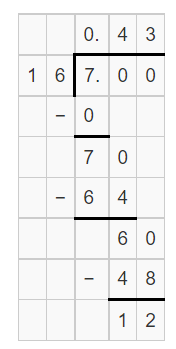Hence, from the above,
We can conclude that
The length of the bolt in the form of a decimal is: 0.43

Question 23.
Astronomy The weight of an object on the moon is $$\frac{1}{6}$$ of its weight on Earth. Write $$\frac{1}{6}$$ as a decimal.
It is given that
The weight of an object on the moon is $$\frac{1}{6}$$ of its weight on Earth
Now,
The given fraction is: $$\frac{1}{6}$$
Now,
By using the Long Division,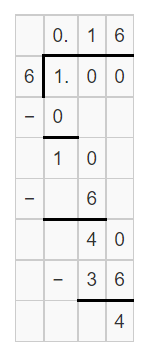Hence, from the above,
We can conclude that
The representation of $$\frac{1}{6}$$ in the form of a decimal is: 0.16

Lesson 1.1 Rational Numbers as Decimals Answer Key Question 24.
The distance to the nearest gas station is 2$$\frac{3}{4}$$ miles. What is this distance written as a decimal?
It is given that
The distance to the nearest gas station is 2$$\frac{3}{4}$$ miles
Now,
The given mixed number is: 2$$\frac{3}{4}$$
Now,
The representation of the given mixed number in the form of a fraction is: $$\frac{11}{4}$$
Now,
By using the Long Division,Hence, from the above,
We can conclude that
The representation of the distance in the form of a decimal is: 2.75

Question 25.
A pitcher on a baseball team has pitched 98$$\frac{2}{3}$$ innings. What is the number of innings written as a decimal?
It is given that
A pitcher on a baseball team has pitched 98$$\frac{2}{3}$$ innings
Now,
The given mixed number is: 98$$\frac{2}{3}$$
Now,
The representation of the given mixed number in the form of a fraction is: $$\frac{296}{3}$$
Now,
By using the Long Division,Hence, from the above,
We can conclude that
The representation of the number of innings in the form of a decimal number is: 98.66

Question 26.
A Coast Guard ship patrols an area of 125 square miles. The area the ship patrols is a square. About how long is each side of the square? Round your answer to the nearest mile.
It is given that
A Coast Guard ship patrols an area of 125 square miles. The area the ship patrols is a square
Now,
We know that,
The area of a square = Side²
So,
Side² = 125
Side = $$\sqrt{125}$$
Now,
We know that,
125 is not a perfect square
Now,
Find the perfect squares that lie between 125
So,
121 < 125 < 144
$$\sqrt{121}$$ < $$\sqrt{125}$$ < $$\sqrt{144}$$
11 < $$\sqrt{125}$$ < 12
Now,
11² = 121
12²= 144
Now,
From the above,
We can observe that
The Estimate of $$\sqrt{125}$$ is approximately equal to: 11
Hence, from the above,
We can conclude that
The length of each side of the square is about 11 miles

Question 27.
Each square on Olivia’s chessboard Is 11 square centimeters. A chessboard has 8 squares on each side. To the nearest tenth, what is the width of Olivia’s chessboard?
It is given that
Each square on Olivia’s chessboard Is 11 square centimeters. A chessboard has 8 squares on each side
Now,
The total area of the chessboard = 8 × 11
= 88 square centimeters
Now,
We know that,
The area of a square = Side²
So,
Side² = 88
Side = ±$$\sqrt{88}$$
Now,
Find the squares that lie between 88
So,
81 < 88 < 100
So,
9 < $$\sqrt{88}$$ < 10
So,
The approximate value of $$\sqrt{88}$$ is: 9
Hence, from the above,
We can conclude that
The width of Olivia’s chessboard is about 9 centimeters

Go Math 8th Grade Lesson 1.1 Question 28.
The thickness of a surfboard relates to the weight of the surfer. A surfboard is 21$$\frac{3}{16}$$ inches wide and 2$$\frac{3}{8}$$ inches thick. Write each dimension as a decimal.
It is given that
The thickness of a surfboard relates to the weight of the surfer. A surfboard is 21$$\frac{3}{16}$$ inches wide and 2$$\frac{3}{8}$$ inches thick.
Now,
The given mixed numbers are: 21$$\frac{3}{16}$$ and 2$$\frac{3}{8}$$
Now,
The representation of the given mixed numbers in the form of fractions are: $$\frac{339}{16}$$ and $$\frac{19}{8}$$
Now,
The representation of the width of the surfboard in the form of a fraction is: $$\frac{339}{16}$$ inches
The representation of the thickness of the surfboard in the form of a fraction is: $$\frac{19}{8}$$ inches
Now,
By using the Long Division,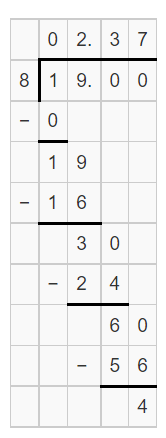Hence, from the above,
We can conclude that
The representation of the width of the surfboard in the form of a decimal is: 21.18
The representation of the thickness of the surfboard in the form of a decimal is: 2.37

Question 29.
A gallon of stain can cover a square deck with an area of 300 square feet. About how long is each side of the deck? Round your answer to the nearest foot.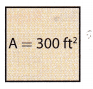It is given that
A gallon of stain can cover a square deck with an area of 300 square feet
Now,
We know that,
The area of a square = Side²
So,
Side² = 300
Side = ±$$\sqrt{300}$$
Now,
Find the squares that lie between 300
So,
289 < 300 < 324
So,
17 < $$\sqrt{300}$$ < 18
So,
The approximate value of $$\sqrt{300}$$ is: 18
Hence, from the above,
We can conclude that
The length of each side of the deck is: 18 feet

Lesson 1.1 Rational Numbers as Decimals Question 30.
The area of a square field is 200 square feet. What is the approximate length of each side of the field? Round your answer to the nearest foot.
It is given that
The area of a square field is 200 square feet.
Now,
We know that,
The area of a square = Side²
So,
Side² = 200
Side = $$\sqrt{200}$$
Now,
Find the squares that lie between 200
So,
196 < 200 < 225
So,
14 < $$\sqrt{200}$$ < 15
So,
The approximate value of $$\sqrt{200}$$ is: 14
Hence, from the above,
We can conclude that
The length of each side of the field is: 14 feet

Question 31.
Measurement A ruler is marked at every $$\frac{3}{16}$$ inches. Do the labeled measurements convert to terminating or repeating decimals?
It is given that
A ruler is marked at every $$\frac{3}{16}$$ inches.
Now,
By using the Long Division,Now,
From the above Long Division,
We can observe that
The given fraction is a non-terminating decimal
Hence, from the above,
We can conclude that
$$\frac{3}{16}$$ is a non-terminating decimal

Question 32.
Multistep A couple wants to install a square mirror that has an area of 500 square inches. To the nearest tenth of an inch, what length of wood trim is needed to go around the mirror?
It is given that
A couple wants to install a square mirror that has an area of 500 square inches
Now,
We know that,
The area of a square = Side²
So,
Side² = 500
Side = $$\sqrt{500}$$
Now,
Find the squares that lie between 500
So,
484 < 500 < 625
So,
24 < $$\sqrt{500}$$ < 25
So,
The approximate value of $$\sqrt{500}$$ is: 24
Hence, from the above,
We can conclude that
The length of wood trim that is needed to go around the mirror is: 24 inches

Rational and Irrational Numbers Grade 8 Question 33.
Multistep A square photo-display board is made up of 60 rows of 60 photos each. The area of each square photo is 4 square inches. How long is each side of the display board?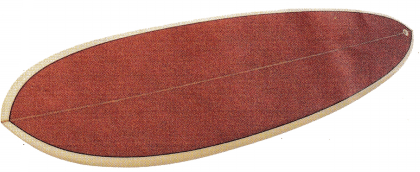It is given that
A square photo-display board is made up of 60 rows of 60 photos each. The area of each square photo is 4 square inches
Now,
The number of photos in each row = (The number of rows) ÷ (The number of photos)
= $$\frac{60}{60}$$
= 1 photo per row
Now,
We know that,
The area of a square = Side²
So,
Side² = 60 × 4
Side² = 240
Side = $$\sqrt{240}$$
Now,
Find the squares that lie between 240
So,
225 < 240 < 256
So,
15 < $$\sqrt{240}$$ < 16
So,
The approximate value of $$\sqrt{500}$$ is: 16
Hence, from the above,
We can conclude that
The length of each side of the display board is: 16 inches

Approximate each irrational number to the nearest 0.05 without using a calculator. Then plot each number on a number line.

Question 34.The given number is: $$\sqrt{24}$$
Now,
Find the perfect squares that is between $$\sqrt{24}$$
So,
4 < $$\sqrt{24}$$ < 5
So,
The value of $$\sqrt{24}$$ lies between 4 and 5
Now,
We know that,
$$\sqrt{24}$$ ≈ 5
So,
The value of $$\sqrt{24}$$ lies closer to: 5
Now,
The representation of the value of $$\sqrt{24}$$ on a number line is:Hence, from the above,
We can conclude that
$$\sqrt{24}$$ is between 4 and 5
So,
$$\sqrt{24}$$ ≈ 5The given number is: $$\sqrt{41}$$
Now,
Find the perfect squares that is between $$\sqrt{41}$$
So,
6 < $$\sqrt{41}$$ < 7
So,
The value of $$\sqrt{41}$$ lies between 6 and 7
Now,
We know that,
$$\sqrt{41}$$ ≈ 6
So,
The value of $$\sqrt{41}$$ lies closer to: 6
Now,
The representation of the value of $$\sqrt{41}$$ on a number line is:Hence, from the above,
We can conclude that
$$\sqrt{41}$$ is between 6 and 7
So,
$$\sqrt{41}$$ ≈ 6

Question 36.
Represent Real-World Problems If every positive number has two square roots and you can find the length of the side of a square window by finding a square root of the area, why is there only one answer for the length of a side?
We know that,
The two square roots of a positive number are:
a) one positive square root
b) one negative square root.
Now,
As long as you’re doing math, on paper, you can work with both of them. But as soon as you start talking about windows or other physical things, it’s pretty silly to talk about a negative length.  When you’re working with real physical things, you usually just ignore the negative square root.
Hence,
There is only one answer for the length of the side

Question 37.
Make a Prediction To find $$\sqrt{5}$$, Beau found 22 = 4 and 32 = 9. He said that since 5 is between 4 and 9, $$\sqrt{5}$$ is between 2 and 3. Beau thinks a good estimate for is $$\sqrt{5}$$ is $$\frac{2+3}{2}$$ = 2.5. Is his estimate high or low? How do you know?
It is given that
To find $$\sqrt{5}$$, Beau found 22 = 4 and 32 = 9. He said that since 5 is between 4 and 9, $$\sqrt{5}$$ is between 2 and 3. Beau thinks a good estimate for is $$\sqrt{5}$$ is $$\frac{2+3}{2}$$ = 2.5
Now,
From the above,
We can observe that
5 is very close to 4
So,
The estimate of $$\sqrt{5}$$ is also close to 2 but not to 3
So,
The estimate of $$\sqrt{5}$$ should be less than 2.5 and greater than 2. i.e.,
2 < Estimate < 2.5
Hence, from the above,
We can conclude that
The Estimate of Beau is high

Texas Go Math Grade 8 Lesson 1.1 H.O.T. Focus On Higher Order Thinking Answer Key

Question 38.
Multistep On a baseball field, the infield area created by the baselines is a square. In a youth baseball league, this area is 3600 square feet. A pony league of younger children uses a smaller baseball field with a distance between each base that is 20 feet less than the youth league. What is the distance between each base for the pony league?
It is given that
On a baseball field, the infield area created by the baselines is a square. In a youth baseball league, this area is 3600 square feet. A pony league of younger children uses a smaller baseball field with a distance between each base that is 20 feet less than the youth league
Now,
We know that,
The area of a square = Side²
So,
The length of each side in a youth baseball league’s field is:
Side² = 3600
Side = $$\sqrt{3600}$$
Side = 60 feet
Now,
The length of each side in a smaller baseball field = 60 – 20
= 40 feet
Hence, from the above,
We can conclude that
The distance between each base for the pony league is: 40 feet

Question 39.
Problem Solving The difference between the square roots of a number is 30. What is the number? Show that your answer is correct.
It is given that
The difference between the square roots of a number is 30
Now,
Let the square root of a number be: x
Now,
We know that,
The square root of a number has 2 numbers. i.e., 1 positive number and 1 negative number
So,
According to the above information,
x – (-x) = 30
x + x = 30
2x = 30
x = $$\frac{30}{2}$$
x = 15
So,
The required number = x²
= 15²
= 225
Hence, from the above,
We can conclude that
The required number is: 225

Lesson 1.1 Write Rational Numbers in Equivalent Forms Answer Key Question 40.
Analyze Relationships If the ratio of the circumference of a circle to its diameter is π, what is the relationship of the circumference to the radius of the circle? Explain.
It is given that
The ratio of the circumference of a circle to its diameter is π
Now,
According to the given information,
$$\frac{Circumference}{Diameter}$$ = π
Now,
We know that,
$$\frac{Circumference}{2 × Radius}$$ = π
$$\frac{Circumference}{Radius}$$ = 2π